Synthetic DivisionCustom SearchSynthetic Division Synthetic division is a shorthand method of dividing a polynomial by a binomial of the form x - a. For example, if 3x4 + 2x3 + 2x2 - x - 6 is to be divided by x - 1, the long form would be as follows: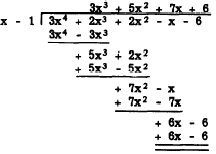Notice that every alternate line of work in this example contains a term which duplicates the one above it. Furthermore, when the subtraction is completed in each step, these duplicated terms cancel each other and thus have no effect on the final result. Another unnecessary duplication results when terms from the dividend are brought down and rewritten prior to subtraction. By omitting these duplications, the work may be condensed as follows: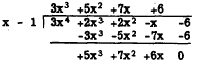The coefficients of the dividend and the constant term of the divisor determine the results of each successive step of multiplication and subtraction. Therefore, we may condense still further by writing only the nonliteral factors, as follows:Notice that if the coefficient of the first term in the dividend is brought down to the last line, then the numbers in the last line are the same as the coefficients of the terms in the quotient. Thus we do not really need to write a separate line of coefficients to represent the quotient. Instead, we bring down the first coefficient of the dividend and make the subtraction "subtotals" serve as coefficients for the rest of the quotient, as follows: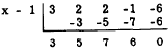The unnecessary writing of plus signs is also eliminated here. The use of synthetic division is limited to divisors of the form x - a, in which the degree of x is 1. Thus the degree of each term in the quotient is 1 less than the degree of the corresponding term in the dividend. The quotient in this example is as follows: 3x3 + 5x2 + 7x + 6 The sequence of operations in synthetic division may be summarized as follows, using as an example the division of 3x - 4x2 + x4 - 3 by x + 2: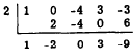First, rearrange the terms of the dividend in descending powers of x. The dividend then becomes x4 - 4x2 + 3x - 3, with 1 understood as the coefficient of the first term. No x3 term appears in the polynomial, but we supply a zero as a place holder for the x3 position. Second, bring down the 1 and multiply it by the +2 of the divisor. Place the result under the zero, and subtract. Multiply the result (-2) by the +2 of the divisor, place the product under the -4 of the dividend, and subtract. Continue this process, finally obtaining x3 - 2x2 + 3 as the quotient. The remainder is -9. Practice problems. In the following problems, perform the indicated operations. In 4, 5, and 6, first use synthetic division and then check your work by long division: 1. (a3 - 3a2 + a) ÷ a 2.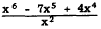3. (10x3 - 7x2y - 16xy2 + 12y3) ÷ (2x2 + xy - 2y2) 4. (x2 + 11x + 30) ÷ (x + 6) 5. (12 + x2 - 7x) ÷ (x - 3) 6. (a2 - 11a + 30) ÷ (a - 5) Answers: 1. a3 - 3a + 1  2. x4 - 7x3 + 4x2  3. 5x - 6y  4. x + 5 5. x - 4 6. a - 6Integrated Publishing, Inc. - A (SDVOSB) Service Disabled Veteran Owned Small Business